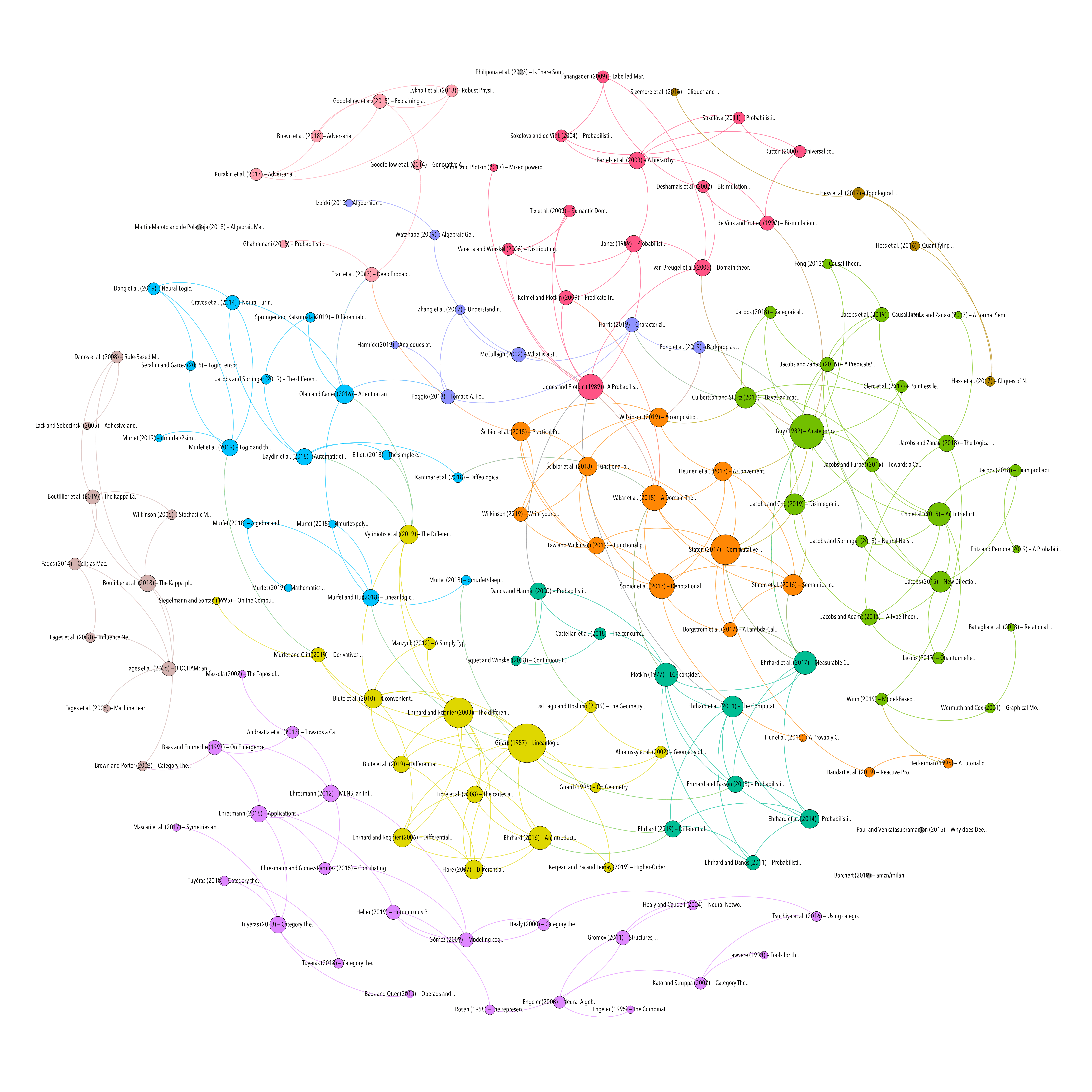MACHINE LEARNING

## Neural Networks, Knowledge and Cognition: A Mathematical Semantic Model Based upon Category Theory

Resource type
Authors/contributors
Title
Neural Networks, Knowledge and Cognition: A Mathematical Semantic Model Based upon Category Theory
Abstract
Category theory can be applied to mathematically model the semantics of cognitive neural systems. We discuss semantics as a hierarchy of concepts, or symbolic descriptions of items sensed and represented in the connection weights distributed throughout a neural network. The hierarchy expresses subconcept relationships, and in a neural network it becomes represented incrementally through a Hebbian-like learning process. The categorical semantic model described here explains the learning process as the derivation of colimits and limits in a concept category. It explains the representation of the concept hierarchy in a neural network at each stage of learning as a system of functors and natural transformations, expressing knowledge coherence across the regions of a multi-regional network equipped with multiple sensors. The model yields design principles that constrain neural network designs capable of the most important aspects of cognitive behavior.
Date
2004
Short Title
Neural Networks, Knowledge and Cognition
Library Catalog
Semantic Scholar
Citation
Healy, M. J., & Caudell, T. P. (2004). Neural Networks, Knowledge and Cognition: A Mathematical Semantic Model Based upon Category Theory.
BIOLOGY, NEUROSCIENCE & PSYCHOLOGY
MACHINE LEARNING
Methodology
Processing time: 0.02 secondsGraph of references (from Zotero to Gephi via Zotnet with this script)